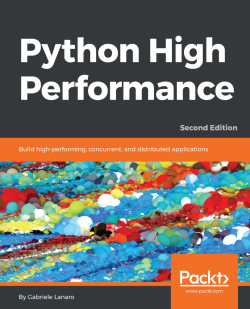# Python High Performance - Second Edition3.8 (5 reviews total)
By Dr. Gabriele Lanaro
• \$33.99 eBook
• \$41.99 Print + eBook
• \$12.99 eBook + Subscription
What do you get with a Packt Subscription?

• Constantly updated with 100+ new titles each month
• Breadth and depth in over 1,000+ technologies

Python is a versatile language that has found applications in many industries. The clean syntax, rich standard library, and vast selection of third-party libraries make Python a wildly popular language.

Python High Performance is a practical guide that shows how to leverage the power of both native and third-party Python libraries to build robust applications.

The book explains how to use various profilers to find performance bottlenecks and apply the correct algorithm to fix them. The reader will learn how to effectively use NumPy and Cython to speed up numerical code. The book explains concepts of concurrent programming and how to implement robust and responsive applications using Reactive programming. Readers will learn how to write code for parallel architectures using Tensorflow and Theano, and use a cluster of computers for large-scale computations using technologies such as Dask and PySpark.

By the end of the book, readers will have learned to achieve performance and scale from their Python applications.

Publication date:
May 2017
Publisher
Packt
Pages
270
ISBN
9781787282896

# Benchmarking and Profiling

Recognizing the slow parts of your program is the single most important task when it comes to speeding up your code. Luckily, in most cases, the code that causes the application to slow down is a very small fraction of the program. By locating those critical sections, you can focus on the parts that need improvement without wasting time in micro-optimization.

Profiling is the technique that allows us to pinpoint the most resource-intensive spots in an application. A profiler is a program that runs an application and monitors how long each function takes to execute, thus detecting the functions in which your application spends most of its time.

Python provides several tools to help us find these bottlenecks and measure important performance metrics. In this chapter, we will learn how to use the standard cProfile module and the line_profiler third-party package. We will also learn how to profile an application's memory consumption through the memory_profiler tool. Another useful tool that we will cover is KCachegrind, which can be used to graphically display the data produced by various profilers.

Benchmarks are small scripts used to assess the total execution time of your application. We will learn how to write benchmarks and how to accurately time your programs.

The list of topics we will cover in this chapter is as follows:

• General principles of high performance programming
• Writing tests and benchmarks
• The Unix time command
• The Python timeit module
• Testing and benchmarking with pytest
• The cProfile standard tool
• Interpreting profiling results with KCachegrind
• line_profiler and memory_profiler tools
• Disassembling Python code through the dis module

When designing a performance-intensive program, the very first step is to write your code without bothering with small optimizations:

"Premature optimization is the root of all evil."
- Donald Knuth

In the early development stages, the design of the program can change quickly and may require large rewrites and reorganizations of the code base. By testing different prototypes without the burden of optimization, you are free to devote your time and energy to ensure that the program produces correct results and that the design is flexible. After all, who needs an application that runs fast but gives the wrong answer?

The mantras that you should remember when optimizing your code are as follows:

• Make it run: We have to get the software in a working state, and ensure that it produces the correct results. This exploratory phase serves to better understand the application and to spot major design issues in the early stages.
• Make it right: We want to ensure that the design of the program is solid. Refactoring should be done before attempting any performance optimization. This really helps separate the application into independent and cohesive units that are easier to maintain.
• Make it fast: Once our program is working and is well structured, we can focus on performance optimization. We may also want to optimize memory usage if that constitutes an issue.

In this section, we will write and profile a particle simulator test application. The simulator is a program that takes some particles and simulates their movement over time according to a set of laws that we impose. These particles can be abstract entities or correspond to physical objects, for example, billiard balls moving on a table, molecules in gas, stars moving through space, smoke particles, fluids in a chamber, and so on.

Computer simulations are useful in fields such as Physics, Chemistry, Astronomy, and many other disciplines. The applications used to simulate systems are particularly performance-intensive and scientists and engineers spend an inordinate amount of time optimizing these codes. In order to study realistic systems, it is often necessary to simulate a very high number of bodies and every small increase in performance counts.

In our first example, we will simulate a system containing particles that constantly rotate around a central point at various speeds, just like the hands of a clock.

The necessary information to run our simulation will be the starting positions of the particles, the speed, and the rotation direction. From these elements, we have to calculate the position of the particle in the next instant of time. An example system is shown in the following figure. The origin of the system is the (0, 0) point, the position is indicated by the x, y vector and the velocity is indicated by the vx, vy vector: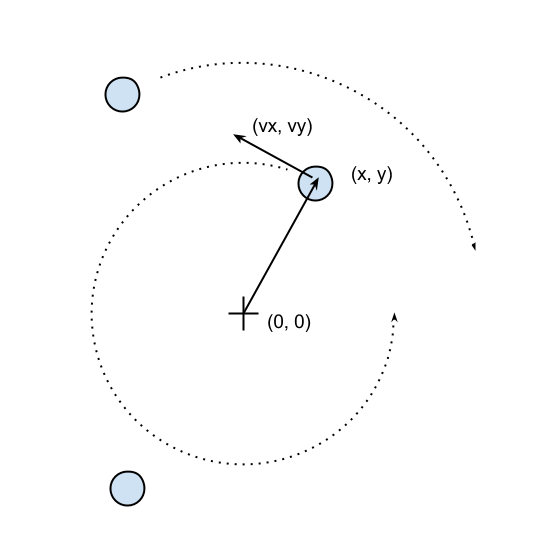The basic feature of a circular motion is that the particles always move perpendicular to the direction connecting the particle and the center. To move the particle, we simply change the position by taking a series of very small steps (which correspond to advancing the system for a small interval of time) in the direction of motion, as shown in the following figure: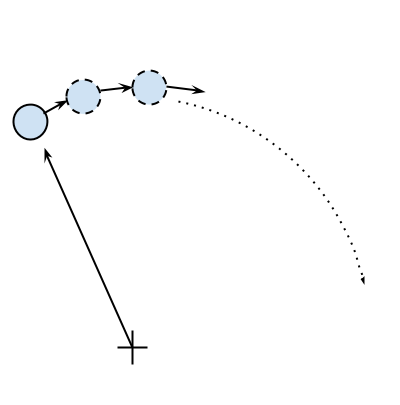We will start by designing the application in an object-oriented way. According to our requirements, it is natural to have a generic Particle class that stores the particle positions, x and y, and their angular velocity, ang_vel:

```    class Particle:         def __init__(self, x, y, ang_vel):             self.x = x             self.y = y             self.ang_vel = ang_vel
```

Note that we accept positive and negative numbers for all the parameters (the sign of ang_vel will simply determine the direction of rotation).

Another class, called ParticleSimulator, will encapsulate the laws of motion and will be responsible for changing the positions of the particles over time. The __init__ method will store a list of Particle instances and the evolve method will change the particle positions according to our laws.

We want the particles to rotate around the position corresponding to the x=0 and y=0 coordinates, at a constant speed. The direction of the particles will always be perpendicular to the direction from the center (refer to the first figure of this chapter). To find the direction of the movement along the x and y axes (corresponding to the Python v_x and v_y variables), it is sufficient to use these formulae:

```    v_x = -y / (x**2 + y**2)**0.5    v_y = x / (x**2 + y**2)**0.5
```

If we let one of our particles move, after a certain time t, it will reach another position following a circular path. We can approximate a circular trajectory by dividing the time interval, t, into tiny time steps, dt, where the particle moves in a straight line tangentially to the circle. The final result is just an approximation of a circular motion. In order to avoid a strong divergence, such as the one illustrated in the following figure, it is necessary to take very small time steps: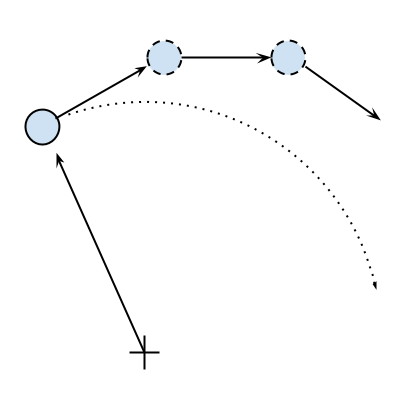In a more schematic way, we have to carry out the following steps to calculate the particle position at time t:

1. Calculate the direction of motion ( v_x and v_y).
2. Calculate the displacement (d_x and d_y), which is the product of time step, angular velocity, and direction of motion.
3. Repeat steps 1 and 2 for enough times to cover the total time t.

The following code shows the full ParticleSimulator implementation:

```    class ParticleSimulator:         def __init__(self, particles):             self.particles = particles         def evolve(self, dt):             timestep = 0.00001             nsteps = int(dt/timestep)                  for i in range(nsteps):                for p in self.particles:                    # 1. calculate the direction                     norm = (p.x**2 + p.y**2)**0.5                     v_x = -p.y/norm                     v_y = p.x/norm                     # 2. calculate the displacement                     d_x = timestep * p.ang_vel * v_x                     d_y = timestep * p.ang_vel * v_y                     p.x += d_x                     p.y += d_y                     # 3. repeat for all the time steps
```

We can use the matplotlib library to visualize our particles. This library is not included in the Python standard library, and it can be easily installed using the pip install matplotlib command.

Alternatively, you can use the Anaconda Python distribution (https://store.continuum.io/cshop/anaconda/) that includes matplotlib and most of the other third-party packages used in this book. Anaconda is free and is available for Linux, Windows, and Mac.

To make an interactive visualization, we will use the matplotlib.pyplot.plot function to display the particles as points and the matplotlib.animation.FuncAnimation class to animate the evolution of the particles over time.

The visualize function takes a particle ParticleSimulator instance as an argument and displays the trajectory in an animated plot. The steps necessary to display the particle trajectory using the matplotlib tools are as follows:

• Set up the axes and use the plot function to display the particles. plot takes a list of x and y coordinates.
• Write an initialization function, init, and a function, animate, that updates the x and y coordinates using the line.set_data method.
• Create a FuncAnimation instance by passing the init and animate functions plus the interval parameters, which specify the update interval, and blit, which improves the update rate of the image.
• Run the animation with plt.show():
```    from matplotlib import pyplot as plt     from matplotlib import animation     def visualize(simulator):         X = [p.x for p in simulator.particles]         Y = [p.y for p in simulator.particles]         fig = plt.figure()         ax = plt.subplot(111, aspect='equal')         line, = ax.plot(X, Y, 'ro')              # Axis limits         plt.xlim(-1, 1)         plt.ylim(-1, 1)         # It will be run when the animation starts         def init():             line.set_data([], [])             return line, # The comma is important!        def animate(i):             # We let the particle evolve for 0.01 time units             simulator.evolve(0.01)             X = [p.x for p in simulator.particles]             Y = [p.y for p in simulator.particles]             line.set_data(X, Y)             return line,         # Call the animate function each 10 ms         anim = animation.FuncAnimation(fig,                                       animate,                                       init_func=init,                                       blit=True,                                       interval=10)         plt.show()
```

To test things out, we define a small function, test_visualize, that animates a system of three particles rotating in different directions. Note that the third particle completes a round three times faster than the others:

```    def test_visualize():         particles = [Particle(0.3, 0.5, 1),                      Particle(0.0, -0.5, -1),                      Particle(-0.1, -0.4, 3)]         simulator = ParticleSimulator(particles)         visualize(simulator)     if __name__ == '__main__':         test_visualize()
```

The test_visualize function is helpful to graphically understand the system time evolution. In the following section, we will write more test functions to properly verify program correctness and measure performance.

# Writing tests and benchmarks

Now that we have a working simulator, we can start measuring our performance and tune-up our code so that the simulator can handle as many particles as possible. As a first step, we will write a test and a benchmark.

We need a test that checks whether the results produced by the simulation are correct or not. Optimizing a program commonly requires employing multiple strategies; as we rewrite our code multiple times, bugs may easily be introduced. A solid test suite ensures that the implementation is correct at every iteration so that we are free to go wild and try different things with the confidence that, if the test suite passes, the code will still work as expected.

Our test will take three particles, simulate them for 0.1 time units, and compare the results with those from a reference implementation. A good way to organize your tests is using a separate function for each different aspect (or unit) of your application. Since our current functionality is included in the evolve method, our function will be named test_evolve. The following code shows the test_evolve implementation. Note that, in this case, we compare floating point numbers up to a certain precision through the fequal function:

```    def test_evolve():         particles = [Particle( 0.3,  0.5, +1),                      Particle( 0.0, -0.5, -1),                      Particle(-0.1, -0.4, +3)]         simulator = ParticleSimulator(particles)         simulator.evolve(0.1)         p0, p1, p2 = particles         def fequal(a, b, eps=1e-5):             return abs(a - b) < eps         assert fequal(p0.x, 0.210269)         assert fequal(p0.y, 0.543863)         assert fequal(p1.x, -0.099334)         assert fequal(p1.y, -0.490034)         assert fequal(p2.x,  0.191358)         assert fequal(p2.y, -0.365227)     if __name__ == '__main__':         test_evolve()
```

A test ensures the correctness of our functionality but gives little information about its running time. A benchmark is a simple and representative use case that can be run to assess the running time of an application. Benchmarks are very useful to keep score of how fast our program is with each new version that we implement.

We can write a representative benchmark by instantiating a thousand Particle objects with random coordinates and angular velocity, and feed them to a ParticleSimulator class. We then let the system evolve for 0.1 time units:

```    from random import uniform     def benchmark():         particles = [Particle(uniform(-1.0, 1.0),                               uniform(-1.0, 1.0),                               uniform(-1.0, 1.0))                       for i in range(1000)]         simulator = ParticleSimulator(particles)         simulator.evolve(0.1)     if __name__ == '__main__':         benchmark()
```

A very simple way to time a benchmark is through the Unix time command. Using the time command, as follows, you can easily measure the execution time of an arbitrary process:

```    \$ time python simul.pyreal    0m1.051suser    0m1.022ssys     0m0.028s
```
The time command is not available for Windows. To install Unix tools, such as time, on Windows you can use the cygwin shell, downloadable from the official website (http://www.cygwin.com/). Alternatively, you can use similar PowerShell commands, such as Measure-Command (https://msdn.microsoft.com/en-us/powershell/reference/5.1/microsoft.powershell.utility/measure-command), to measure execution time.

By default, time displays three metrics:

• real: The actual time spent running the process from start to finish, as if it was measured by a human with a stopwatch
• user: The cumulative time spent by all the CPUs during the computation
• sys: The cumulative time spent by all the CPUs during system-related tasks, such as memory allocation

Note that sometimes user + sys might be greater than real, as multiple processors may work in parallel.

time also offers richer formatting options. For an overview, you can explore its manual (using the man time command). If you want a summary of all the metrics available, you can use the -v option.

The Unix time command is one of the simplest and more direct ways to benchmark a program. For an accurate measurement, the benchmark should be designed to have a long enough execution time (in the order of seconds) so that the setup and tear-down of the process is small compared to the execution time of the application. The user metric is suitable as a monitor for the CPU performance, while the real metric also includes the time spent in other processes while waiting for I/O operations.

Another convenient way to time Python scripts is the timeit module. This module runs a snippet of code in a loop for n times and measures the total execution times. Then, it repeats the same operation r times (by default, the value of r is 3) and records the time of the best run. Due to this timing scheme, timeit is an appropriate tool to accurately time small statements in isolation.

The timeit module can be used as a Python package, from the command line or from IPython.

IPython is a Python shell design that improves the interactivity of the Python interpreter. It boosts tab completion and many utilities to time, profile, and debug your code. We will use this shell to try out snippets throughout the book. The IPython shell accepts magic commands--statements that start with a % symbol--that enhance the shell with special behaviors. Commands that start with %% are called cell magics, which can be applied on multi-line snippets (termed as cells).

IPython is available on most Linux distributions through pip and is included in Anaconda.

You can use IPython as a regular Python shell (ipython), but it is also available in a Qt-based version (ipython qtconsole) and as a powerful browser-based interface (jupyter notebook).

In IPython and command-line interfaces, it is possible to specify the number of loops or repetitions with the -n and -r options. If not specified, they will be automatically inferred by timeit. When invoking timeit from the command line, you can also pass some setup code, through the -s option, which will execute before the benchmark. In the following snippet, the IPython command line and Python module version of timeit are demonstrated:

```# IPython Interface \$ ipython In : from simul import benchmark In : %timeit benchmark() 1 loops, best of 3: 782 ms per loop # Command Line Interface \$ python -m timeit -s 'from simul import benchmark' 'benchmark()'10 loops, best of 3: 826 msec per loop # Python Interface # put this function into the simul.py script import timeitresult = timeit.timeit('benchmark()', setup='from __main__ import benchmark', number=10)# result is the time (in seconds) to run the whole loop result = timeit.repeat('benchmark()', setup='from __main__ import benchmark', number=10, repeat=3) # result is a list containing the time of each repetition (repeat=3 in this case)
```

Note that while the command line and IPython interfaces automatically infer a reasonable number of loops n, the Python interface requires you to explicitly specify a value through the number argument.

# Better tests and benchmarks with pytest-benchmark

The Unix time command is a versatile tool that can be used to assess the running time of small programs on a variety of platforms. For larger Python applications and libraries, a more comprehensive solution that deals with both testing and benchmarking is pytest, in combination with its pytest-benchmark plugin.

In this section, we will write a simple benchmark for our application using the pytest testing framework. For the interested reader, the pytest documentation, which can be found at http://doc.pytest.org/en/latest/, is the best resource to learn more about the framework and its uses.

You can install pytest from the console using the pip install pytest command. The benchmarking plugin can be installed, similarly, by issuing the pip install pytest-benchmark command.

A testing framework is a set of tools that simplifies writing, executing, and debugging tests and provides rich reports and summaries of the test results. When using the pytest framework, it is recommended to place tests separately from the application code. In the following example, we create the test_simul.py file, which contains the test_evolve function:

```    from simul import Particle, ParticleSimulator    def test_evolve():        particles = [Particle( 0.3,  0.5, +1),                     Particle( 0.0, -0.5, -1),                     Particle(-0.1, -0.4, +3)]        simulator = ParticleSimulator(particles)        simulator.evolve(0.1)            p0, p1, p2 = particles        def fequal(a, b, eps=1e-5):            return abs(a - b) < eps        assert fequal(p0.x, 0.210269)        assert fequal(p0.y, 0.543863)        assert fequal(p1.x, -0.099334)        assert fequal(p1.y, -0.490034)        assert fequal(p2.x,  0.191358)        assert fequal(p2.y, -0.365227)
```

The pytest executable can be used from the command line to discover and run tests contained in Python modules. To execute a specific test, we can use the pytest path/to/module.py::function_name syntax. To execute test_evolve, we can type the following command in a console to obtain simple but informative output:

```\$ pytest test_simul.py::test_evolveplatform linux -- Python 3.5.2, pytest-3.0.5, py-1.4.32, pluggy-0.4.0rootdir: /home/gabriele/workspace/hiperf/chapter1, inifile: plugins:collected 2 items test_simul.py .=========================== 1 passed in 0.43 seconds ===========================
```

Once we have a test in place, it is possible for you to execute your test as a benchmark using the pytest-benchmark plugin. If we change our test function so that it accepts an argument named benchmark, the pytest framework will automatically pass the benchmark resource as an argument (in pytest terminology, these resources are called fixtures). The benchmark resource can be called by passing the function that we intend to benchmark as the first argument, followed by the additional arguments. In the following snippet, we illustrate the edits necessary to benchmark the ParticleSimulator.evolve function:

```    from simul import Particle, ParticleSimulator    def test_evolve(benchmark):        # ... previous code        benchmark(simulator.evolve, 0.1)
```

To run the benchmark, it is sufficient to rerun the pytest test_simul.py::test_evolve command. The resulting output will contain detailed timing information regarding the test_evolve function, as shown: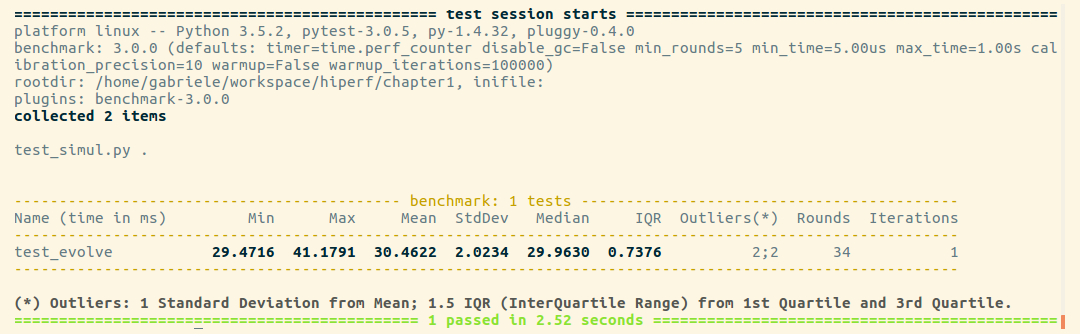For each test collected, pytest-benchmark will execute the benchmark function several times and provide a statistic summary of its running time. The output shown earlier is very interesting as it shows how running times vary between runs.
In this example, the benchmark in test_evolve was run 34 times (column Rounds), its timings ranged between 29 and 41 ms (Min and Max), and the Average and Median times were fairly similar at about 30 ms, which is actually very close to the best timing obtained. This example demonstrates how there can be substantial performance variability between runs, and that when taking timings with one-shot tools such as time, it is a good idea to run the program multiple times and record a representative value, such as the minimum or the median.

pytest-benchmark has many more features and options that can be used to take accurate timings and analyze the results. For more information, consult the documentation at http://pytest-benchmark.readthedocs.io/en/stable/usage.html.

# Finding bottlenecks with cProfile

After assessing the correctness and timing the execution time of the program, we are ready to identify the parts of the code that need to be tuned for performance. Those parts are typically quite small compared to the size of the program.

Two profiling modules are available through the Python standard library:

• The profile module: This module is written in pure Python and adds a significant overhead to the program execution. Its presence in the standard library is because of its vast platform support and because it is easier to extend.
• The cProfile module: This is the main profiling module, with an interface equivalent to profile. It is written in C, has a small overhead, and is suitable as a general purpose profiler.

The cProfile module can be used in three different ways:

• From the command line
• As a Python module
• With IPython

cProfile does not require any change in the source code and can be executed directly on an existing Python script or function. You can use cProfile from the command line in this way:

```\$ python -m cProfile simul.py
```

This will print a long output containing several profiling metrics of all of the functions called in the application. You can use the -s option to sort the output by a specific metric. In the following snippet ,the output is sorted by the tottime metric, which will be described here:

```\$ python -m cProfile -s tottime simul.py
```

The data produced by cProfile can be saved in an output file by passing the -o option. The format that cProfile uses is readable by the stats module and other tools. The usage of the -o option is as follows:

```\$ python -m cProfile -o prof.out simul.py
```

The usage of cProfile as a Python module requires invoking the cProfile.run function in the following way:

```    from simul import benchmark    import cProfile    cProfile.run("benchmark()")
```

You can also wrap a section of code between method calls of a cProfile.Profile object, as shown:

```    from simul import benchmark    import cProfile    pr = cProfile.Profile()    pr.enable()    benchmark()    pr.disable()    pr.print_stats()
```

cProfile can also be used interactively with IPython. The %prun magic command lets you profile an individual function call, as illustrated: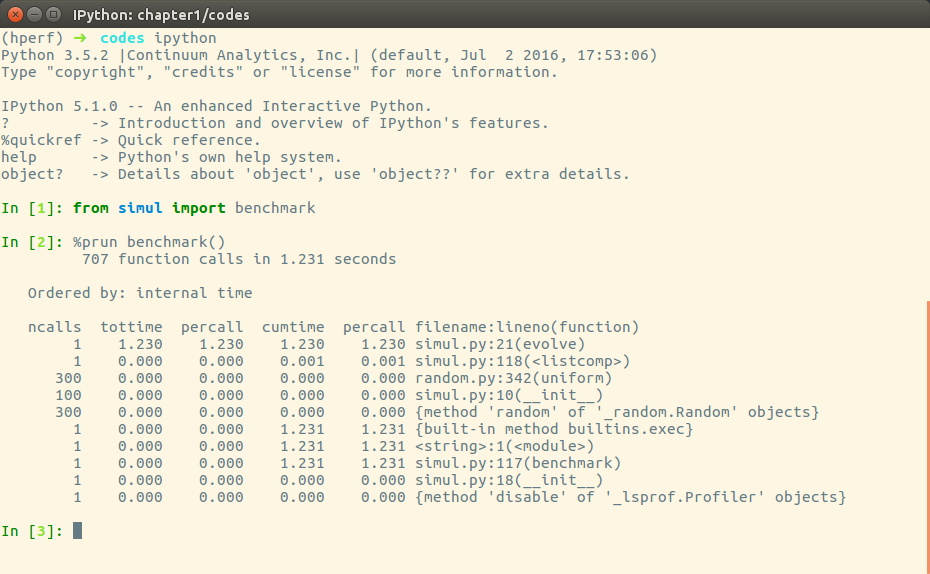The cProfile output is divided into five columns:

• ncalls: The number of times the function was called.
• tottime: The total time spent in the function without taking into account the calls to other functions.
• cumtime: The time in the function including other function calls.
• percall: The time spent for a single call of the function--it can be obtained by dividing the total or cumulative time by the number of calls.
• filename:lineno: The filename and corresponding line numbers. This information is not available when calling C extensions modules.

The most important metric is tottime, the actual time spent in the function body excluding subcalls, which tell us exactly where the bottleneck is.

Unsurprisingly, the largest portion of time is spent in the evolve function. We can imagine that the loop is the section of the code that needs performance tuning.
cProfile only provides information at the function level and does not tell us which specific statements are responsible for the bottleneck. Fortunately, as we will see in the next section, the line_profiler tool is capable of providing line-by-line information of the time spent in the function.

Analyzing the cProfile text output can be daunting for big programs with a lot of calls and subcalls. Some visual tools aid the task by improving navigation with an interactive, graphical interface.

KCachegrind is a Graphical User Interface (GUI) useful to analyze the profiling output emitted by cProfile.

KCachegrind is available in the Ubuntu 16.04 official repositories. The Qt port, QCacheGrind, can be downloaded for Windows from http://sourceforge.net/projects/qcachegrindwin/. Mac users can compile QCacheGrind using Mac Ports (http://www.macports.org/) by following the instructions present in the blog post at http://blogs.perl.org/users/rurban/2013/04/install-kachegrind-on-macosx-with-ports.html.

KCachegrind can't directly read the output files produced by cProfile. Luckily, the pyprof2calltree third-party Python module is able to convert the cProfile output file into a format readable by KCachegrind.

You can install pyprof2calltree from the Python Package Index using the command pip install pyprof2calltree.

To best show the KCachegrind features, we will use another example with a more diversified structure. We define a recursive function, factorial, and two other functions that use factorial, named taylor_exp and taylor_sin. They represent the polynomial coefficients of the Taylor approximations of exp(x) and sin(x):

```    def factorial(n):         if n == 0:             return 1.0         else:             return n * factorial(n-1)     def taylor_exp(n):         return [1.0/factorial(i) for i in range(n)]     def taylor_sin(n):         res = []         for i in range(n):             if i % 2 == 1:                res.append((-1)**((i-1)/2)/float(factorial(i)))             else:                res.append(0.0)         return res     def benchmark():         taylor_exp(500)         taylor_sin(500)     if __name__ == '__main__':         benchmark()
```

To access profile information, we first need to generate the cProfile output file:

```\$ python -m cProfile -o prof.out taylor.py
```

Then, we can convert the output file with pyprof2calltree and launch KCachegrind:

```\$ pyprof2calltree -i prof.out -o prof.calltree\$ kcachegrind prof.calltree # or qcachegrind prof.calltree
```

The output is shown in the following screenshot: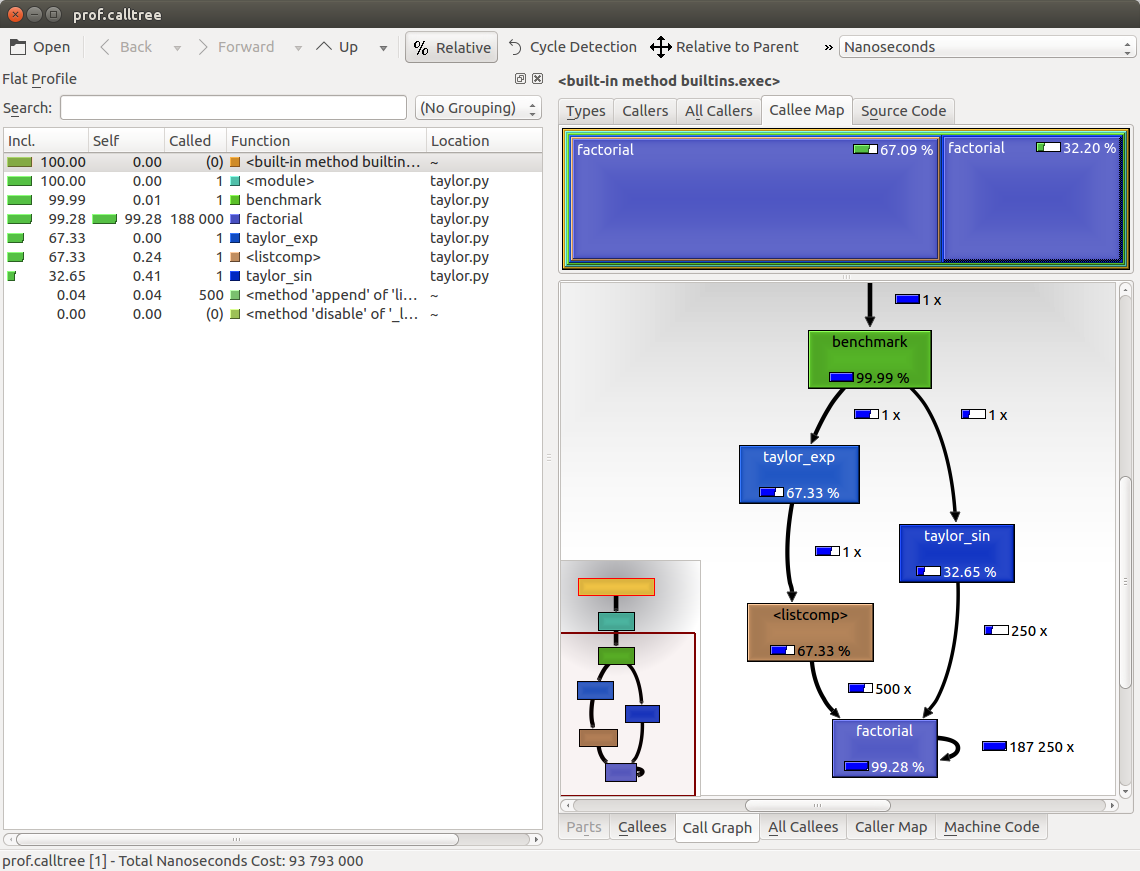The preceding screenshot shows the KCachegrind user interface. On the left, we have an output fairly similar to cProfile. The actual column names are slightly different: Incl. translates to cProfile module's cumtime and Self translates to tottime. The values are given in percentages by clicking on the Relative button on the menu bar. By clicking on the column headers, you can sort them by the corresponding property.

On the top right, a click on the Callee Map tab will display a diagram of the function costs. In the diagram, the time percentage spent by the function is proportional to the area of the rectangle. Rectangles can contain sub-rectangles that represent subcalls to other functions. In this case, we can easily see that there are two rectangles for the factorial function. The one on the left corresponds to the calls made by taylor_exp and the one on the right to the calls made by taylor_sin.

On the bottom right, you can display another diagram, the call graph, by clicking on the Call Graph tab. A call graph is a graphical representation of the calling relationship between the functions; each square represents a function and the arrows imply a calling relationship. For example, taylor_exp calls factorial 500 times, and taylor_sin calls factorial 250 times. KCachegrind also detects recursive calls: factorial calls itself 187250 times.

You can navigate to the Call Graph or the Caller Map tab by double-clicking on the rectangles; the interface will update accordingly, showing that the timing properties are relative to the selected function. For example, double-clicking on taylor_exp will cause the graph to change, showing only the contribution of taylor_exp to the total cost.

Gprof2Dot (https://github.com/jrfonseca/gprof2dot) is another popular tool used to produce call graphs. Starting from output files produced by one of the supported profilers, it will generate a .dot diagram representing the call graph.

# Profile line by line with line_profiler

Now that we know which function we have to optimize, we can use the line_profiler module that provides information on how time is spent in a line-by-line fashion. This is very useful in situations where it's difficult to determine which statements are costly. The line_profiler module is a third-party module that is available on the Python Package Index and can be installed by following the instructions at https://github.com/rkern/line_profiler.

In order to use line_profiler, we need to apply a @profile decorator to the functions we intend to monitor. Note that you don't have to import the profile function from another module as it gets injected in the global namespace when running the kernprof.py profiling script. To produce profiling output for our program, we need to add the @profile decorator to the evolve function:

```    @profile     def evolve(self, dt):         # code
```

The kernprof.py script will produce an output file and will print the result of the profiling on the standard output. We should run the script with two options:

• -l to use the line_profiler function
• -v to immediately print the results on screen

The usage of kernprof.py is illustrated in the following line of code:

```\$ kernprof.py -l -v simul.py
```

It is also possible to run the profiler in an IPython shell for interactive editing. You should first load the line_profiler extension that will provide the lprun magic command. Using that command, you can avoid adding the @profile decorator: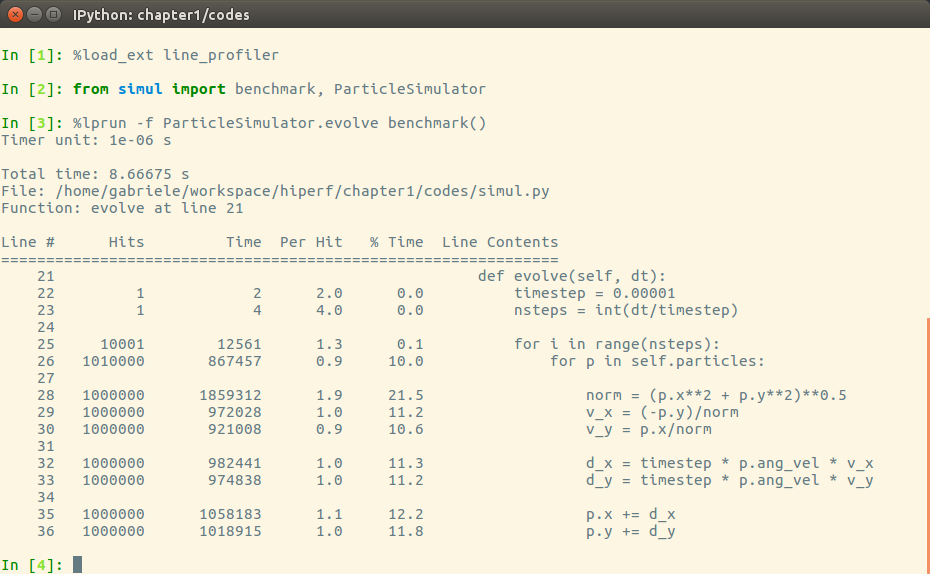The output is quite intuitive and is divided into six columns:

• Line #: The number of the line that was run
• Hits: The number of times that line was run
• Time: The execution time of the line in microseconds (Time)
• Per Hit: Time/hits
• % Time: Fraction of the total time spent executing that line
• Line Contents: The content of the line

By looking at the percentage column, we can get a pretty good idea of where the time is spent. In this case, there are a few statements in the for loop body with a cost of around 10-20 percent each.

# Optimizing our code

Now that we have identified where exactly our application is spending most of its time, we can make some changes and assess the change in performance.

There are different ways to tune up our pure Python code. The way that produces the most remarkable results is to improve the algorithms used. In this case, instead of calculating the velocity and adding small steps, it will be more efficient (and correct as it is not an approximation) to express the equations of motion in terms of radius, r, and angle, alpha, (instead of x and y), and then calculate the points on a circle using the following equation:

```    x = r * cos(alpha)     y = r * sin(alpha)
```

Another way lies in minimizing the number of instructions. For example, we can precalculate the timestep * p.ang_vel factor that doesn't change with time. We can exchange the loop order (first we iterate on particles, then we iterate on time steps) and put the calculation of the factor outside the loop on the particles.

The line-by-line profiling also showed that even simple assignment operations can take a considerable amount of time. For example, the following statement takes more than 10 percent of the total time:

```    v_x = (-p.y)/norm
```

We can improve the performance of the loop by reducing the number of assignment operations performed. To do that, we can avoid intermediate variables by rewriting the expression into a single, slightly more complex statement (note that the right-hand side gets evaluated completely before being assigned to the variables):

```    p.x, p.y = p.x - t_x_ang*p.y/norm, p.y + t_x_ang * p.x/norm
```

This leads to the following code:

```        def evolve_fast(self, dt):             timestep = 0.00001             nsteps = int(dt/timestep)             # Loop order is changed             for p in self.particles:                 t_x_ang = timestep * p.ang_vel                 for i in range(nsteps):                     norm = (p.x**2 + p.y**2)**0.5                     p.x, p.y = (p.x - t_x_ang * p.y/norm,                                p.y + t_x_ang * p.x/norm)
```

After applying the changes, we should verify that the result is still the same by running our test. We can then compare the execution times using our benchmark:

```\$ time python simul.py # Performance Tunedreal    0m0.756suser    0m0.714ssys    0m0.036s\$ time python simul.py # Originalreal    0m0.863suser    0m0.831ssys    0m0.028s
```

As you can see, we obtained only a modest increment in speed by making a pure Python micro-optimization.

# The dis module

Sometimes it's not easy to estimate how many operations a Python statement will take. In this section, we will dig into the Python internals to estimate the performance of individual statements. In the CPython interpreter, Python code is first converted to an intermediate representation, the bytecode, and then executed by the Python interpreter.

To inspect how the code is converted to bytecode, we can use the dis Python module (dis stands for disassemble). Its usage is really simple; all that is needed is to call the dis.dis function on the ParticleSimulator.evolve method:

```    import dis     from simul import ParticleSimulator     dis.dis(ParticleSimulator.evolve)
```

This will print, for each line in the function, a list of bytecode instructions. For example, the v_x = (-p.y)/norm statement is expanded in the following set of instructions:

```    29           85 LOAD_FAST                5 (p)                  88 LOAD_ATTR                4 (y)                  91 UNARY_NEGATIVE                         92 LOAD_FAST                6 (norm)                  95 BINARY_TRUE_DIVIDE                     96 STORE_FAST               7 (v_x)
```

LOAD_FAST loads a reference of the p variable onto the stack and LOAD_ATTR loads the y attribute of the item present on top of the stack. The other instructions, UNARY_NEGATIVE and BINARY_TRUE_DIVIDE, simply do arithmetic operations on top-of-stack items. Finally, the result is stored in v_x (STORE_FAST).

By analyzing the dis output, we can see that the first version of the loop produces 51 bytecode instructions while the second gets converted into 35 instructions.

The dis module helps discover how the statements get converted and serves mainly as an exploration and learning tool of the Python bytecode representation.

To improve our performance even further, we can keep trying to figure out other approaches to reduce the amount of instructions. It's clear, however, that this approach is ultimately limited by the speed of the Python interpreter and it is probably not the right tool for the job. In the following chapters, we will see how to speed up interpreter-limited calculations by executing fast specialized versions written in a lower level language (such as C or Fortran).

# Profiling memory usage with memory_profiler

In some cases, high memory usage constitutes an issue. For example, if we want to handle a huge number of particles, we will incur a memory overhead due to the creation of many Particle instances.

The memory_profiler module summarizes, in a way similar to line_profiler, the memory usage of the process.

The memory_profiler package is also available on the Python Package Index. You should also install the psutil module (https://github.com/giampaolo/psutil) as an optional dependency that will make memory_profiler considerably faster.

Just like line_profiler, memory_profiler also requires the instrumentation of the source code by placing a @profile decorator on the function we intend to monitor. In our case, we want to analyze the benchmark function.

We can slightly change benchmark to instantiate a considerable amount (100000) of Particle instances and decrease the simulation time:

```    def benchmark_memory():         particles = [Particle(uniform(-1.0, 1.0),                               uniform(-1.0, 1.0),                               uniform(-1.0, 1.0))                       for i in range(100000)]         simulator = ParticleSimulator(particles)         simulator.evolve(0.001)
```

We can use memory_profiler from an IPython shell through the %mprun magic command as shown in the following screenshot: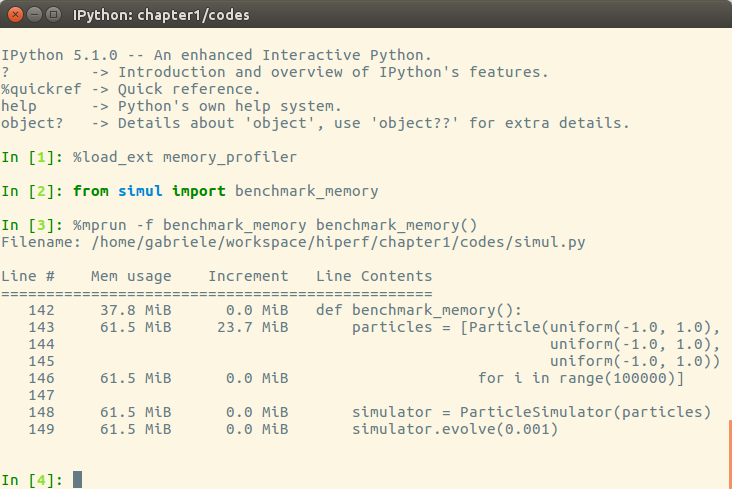It is possible to run memory_profiler from the shell using the mprof run command after adding the @profile decorator.

From the Increment column, we can see that 100,000 Particle objects take 23.7 MiB of memory.

1 MiB (mebibyte) is equivalent to 1,048,576 bytes. It is different from 1 MB (megabyte), which is equivalent to 1,000,000 bytes.

We can use __slots__ on the Particle class to reduce its memory footprint. This feature saves some memory by avoiding storing the variables of the instance in an internal dictionary. This strategy, however, has a drawback--it prevents the addition of attributes other than the ones specified in __slots__ :

```    class Particle:        __slots__ = ('x', 'y', 'ang_vel')         def __init__(self, x, y, ang_vel):             self.x = x             self.y = y             self.ang_vel = ang_vel
```

We can now rerun our benchmark to assess the change in memory consumption, the result is displayed in the following screenshot: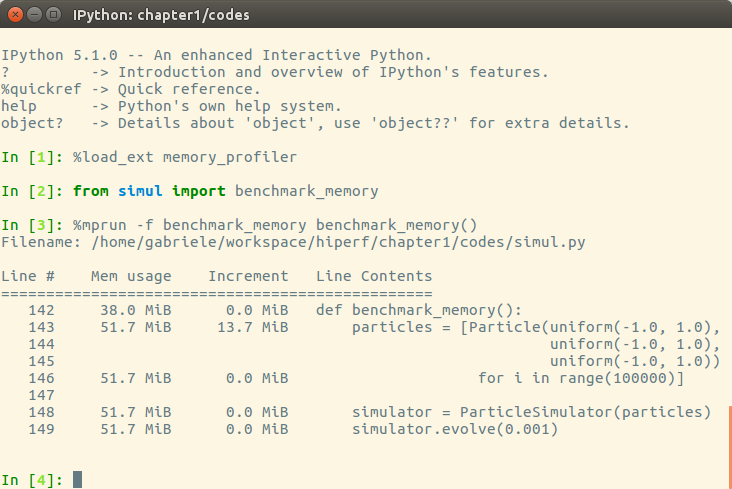By rewriting the Particle class using __slots__, we can save about 10 MiB of memory.

# Summary

In this chapter, we introduced the basic principles of optimization and applied those principles to a test application. When optimizing, the first thing to do is test and identify the bottlenecks in the application. We saw how to write and time a benchmark using the time Unix command, the Python timeit module, and the full-fledged pytest-benchmark package. We learned how to profile our application using cProfile, line_profiler, and memory_profiler, and how to analyze and navigate the profiling data graphically with KCachegrind.

In the next chapter, we will explore how to improve performance using algorithms and data structures available in the Python standard library. We will cover scaling, sample usage of several data structures, and learn techniques such as caching and memoization.